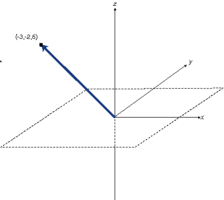## Standards as axiomsAn important, nay, foundational part of my mental model for how Internet scale systems work (and many other things, in fact), is that I view standards as axioms.

In linear algebra, there’s the concept of span, which is, effectively, a function that takes a set of vectors as input, and yields the vector space spanned by those vectors; the set of all reachable points. Also, for any given vector space you can find a set of axioms – a minimal set of vectors which are linearly independent of each other (orthogonal), but still span the space (note; I use “axioms” here to refer to a normalized set of basis vectors).

So given, say, HTTP and URIs as axioms (because they’re independent), I can picture the space reachable using those axioms, which is the set of all tasks that can be coordinated without any additional (beyond the axioms) a priori agreement; in this case, the ability to exchange data between untrusted parties over the Internet. I can also easily add other axioms to the fold and envision how the space expands, so I can understand what adding the new axiom buys me. For example, I can understand what adding RDF to the Web gives me.

More interestingly (though far more difficult – entropy sucks), I can work backwards by imagining how I want the space to look, then figure out what axiom – what new pervasively deployed standard – would give me the desired result.

As mentioned, I try to evaluate many things this way, and at least where I know enough to be able to (even roughly) identify the axioms. It’s why Web services first set off my bunk-o-meter, because treating HTTP as a transport protocol is akin to replacing my HTTP axiom with a TCP axiom, which severely shrinks the set of possible things that can be coordinated … to the empty set, in fact. Oops!